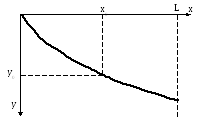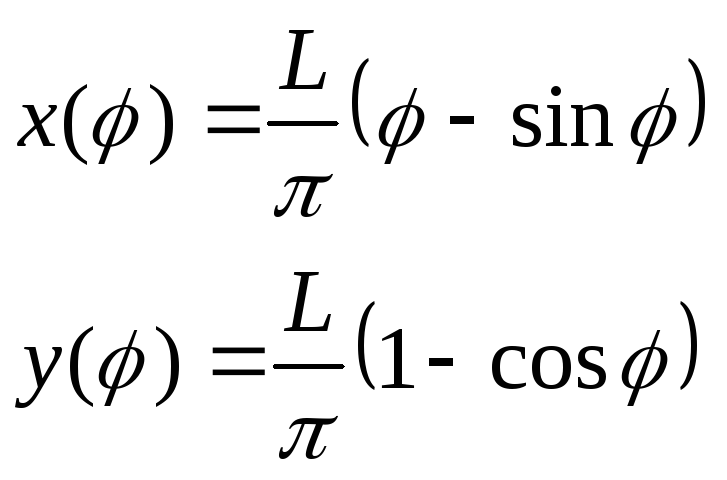# Homework # 1; due Tuesday, Sep

 Page 1/3 Date 09.02.2019 Size 83 Kb. #80383
1   2   3

Physics H7A Fall 2003 D. Budker

## Homework # 1; due Tuesday, Sept. 2

1. The latitude of San Francisco is approximately 38º North, and that of Moscow is approximately 55º North. If you are on a non-stop flight between these cities, at some point you, most likely, will be flying above the polar circle (66º North), and sometimes nearly over the North Pole. Why is such a route chosen by the airlines?

1. Destroyer ship “Aurora” is heading South-East at a constant speed of 30 knots. At noon, passenger yacht “Pegasus” is found 200 nautical miles directly south of “Aurora” heading North-East at 20 knots.

1. Assuming both ships maintain their heading and speed, determine the time at which the separation between them is minimal.

2. What is this minimal separation?

3. Discuss whether the approximation that the Earth is (locally) flat is adequate for this problem.

Hints: There is a clever trick (that was known to Galileo) that allows solving this problem in a straightforward way. You might have to look up definitions of a nautical mile and a knot.

1. Short exercises from K&K: 1.1, 1.2, 1.4, 1.5, 1.7

## Homework # 2; due Tuesday, Sept. 9

1. Watch the NASA “educational” video found at: http://space.about.com/library/weekly/blvidgalileopisa.htm describing Galileo’s famous leaning tower of Pisa experiment. How many errors can you find in the narration? List the errors and suggest corrections.

1. The most accurate atomic clocks today (for example, the one at the National Institute of Standards and Technology at Boulder, CO) incorporate what’s called an atomic fountain, a device in which atoms that have been laser-cooled to near-zero temperatures are tossed vertically in a vacuum chamber. They proceed to fly up, but eventually turn around and fall due to gravity. To make the clock as accurate as possible, it is desirable to maximize the time the atoms spend in their ballistic journey (I will be happy to explain why this is, but this is a bit outside of H7A).

1. Approximately how long does it take the atoms to return to the bottom of the apparatus, if the height of the vacuum chamber is 3 m?

2. The NIST clock uses cesium (Cs) atoms with atomic weight of 133. What would be the return time for rubidium (Rb) atoms with molecular weight 87?

3. Why do people talk about setting up atomic fountain clocks in space?

1. K&K problems: 1.11, 1.13, 1.14, 1.16, 1.18 (note: since acceleration is a vector quantity, so is jerk. Find the vector expression for jerk.), 1.21

2. Consider a point on the surface of a rigid wheel of radius R rolling on the ground with speed v (without sliding). Find analytical expressions for x(t), y(t), y(x), vx(t), and vx(t) for this point and sketch the plots of these quantities. Note that the y(x) plot represents the trajectory of the point. For the plots representing time dependences, it is convenient to place them one above the other, so they all have a common time axis. This helps visualize what’s going on at any given time. Note: if you prefer to express the trajectory as x(y)instead of y(x), this is OK!

3. Please feel free to give the Instructor and the GSI feedback on homework. Is there too much or too little of it? Are the problems too hard or too easy?

## Homework # 3; due Tuesday, Sept. 16

1. The radius of Mars is about two times smaller than that of the Earth. Using this fact, what can you say about its mass of the red planet and the acceleration due to gravity at the planet’s surface (compared to these quantities for the Earth)? What additional assumptions have you used for these estimates?

2. K&K problems: 2.4, 2.8, 2.10, 2.17, 2.18, 2.22, 2.26, 2.27

## Homework # 4; due Tuesday, Sept. 23

1. A fisherman is sitting at the stern of a boat, and both the boat and the man are at rest. The fishermen than moves towards the bow, and eventually, the boat and the man are at rest again. Find the displacement of the boat assuming that the motion of the boat in the water is turbulent, i.e., it is characterized by a friction force that is -v2, where v is the velocity of the boat with respect to water. You may assume some simple model for the process if necessary (for example, the man jumps, and after a bit of a ballistic flight, lands on the bow).

2. K&K problems: 3.2, 3.4, 3.7, 3.9, 3.12, 3.14, 3.15, 3.20(optional, extra credit)

## Homework # 5; due Tuesday, Sept. 30

1. (Extra credit) It is known that the Coriolis forces are important in atmospheric phenomena such as typhoons, affecting the direction of rotation in the atmospheric vortices depending on the hemisphere and the direction of the vortices’ motion. It has been also suggested that Coriolis forces may be affecting the direction of rotation in the familiar bathtub vortices appearing when you pull the bathtub plug. In this problem, you are asked to estimate the order of magnitude of the Coriolis forces in a bathtub, and argue whether or not they are indeed important.

• Consider an element of water in the bathtub. Estimate its velocity. Is it the same for all elements of water? Which elements are important in this problem?

• Using the angular velocity of the Earth’s rotation and the results of part a), estimate the magnitude of the Coriolis acceleration.

• In order to decide whether the Coriolis forces are important or not, we need to compare them to some other forces acting on water elements. Let us consider just one of such forces (feel free to suggest others!): the force due to imperfect shape of the bathtub. Indeed, if we imagine water going down a curled hose, then, certainly, water will be rotating in the direction given by the shape of the curl. It is hard to imagine that a bathtub would be manufactured without defects at a level of at least 1 mm/per 1 m of the tub length. Estimate the asymmetric accelerations due to these imperfections. How do they compare to the Coriolis acceleration?

• Regardless of the Coriolis forces, can you explain why the bathtub vortices are formed?

1. (Extra credit) A Foucault pendulum is used to demonstrate the fact that the Earth rotates around its axis: the pendulum maintains the plane in which it oscillates, so the oscillation plane is rotating with respect to the room where it is set up (as the room is affixed to the Earth). There is a nice Foucault pendulum that can be seen at the California Academy of Sciences: http://www.calacademy.org/products/pendulum.html. Determine the period of the apparent rotation of the oscillation plane for this particular pendulum.

1. (Extra credit) In the fourth problem of HW-2, you have derived equations describing the trajectory of a point on the surface of a rolling wheel, the cycloid. Let us parameterize a portion of the cycloid in the following way:, where we have placed the beginning of the curve in the origin,